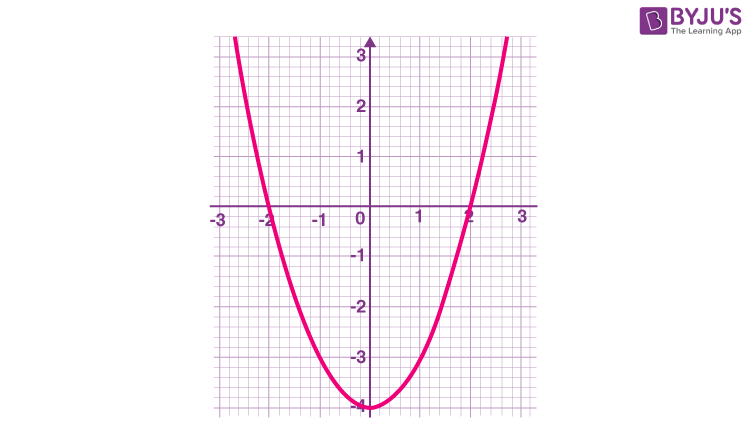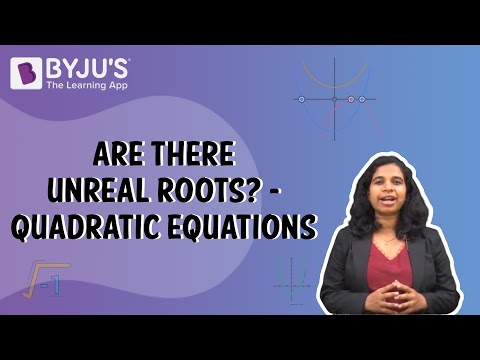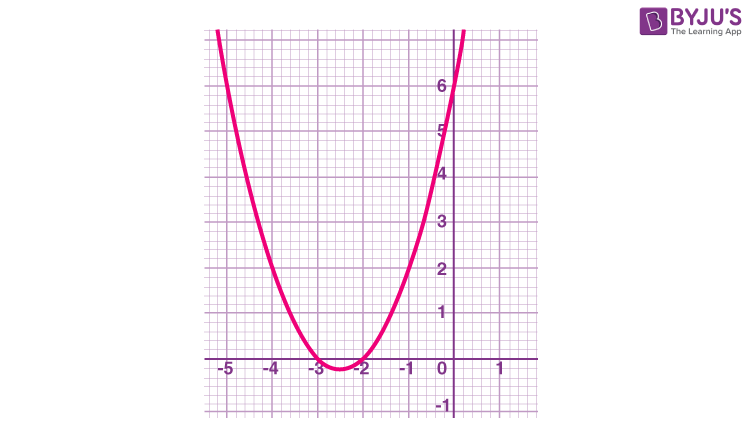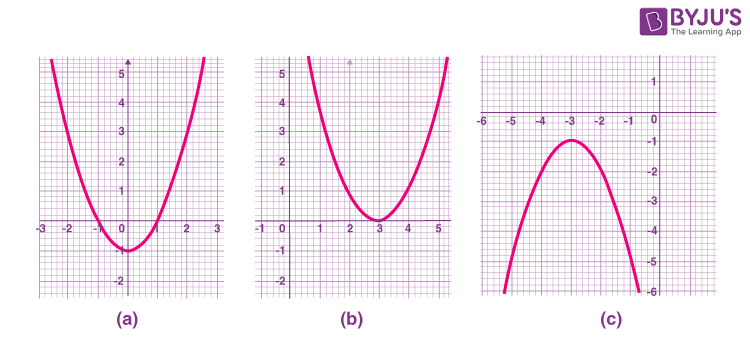# Quadratic Equation Class 10 Notes Chapter 4

## CBSE Class 10 Maths Quadratic Equation Notes:-

Get the complete concepts covered in quadratic equations for Class 10 Maths here. These quadratic equations notes help the students to recall the important definitions, formulas and tricks to solve the problems in the CBSE Board Exams 2023-24. In this article, you will learn the concept of quadratic equations, standard form, nature of roots, and methods for finding the solution for the given quadratic equations with more examples.

A polynomial of the form ax2+bx+c, where a, b and c are real numbers and a0 is called a quadratic polynomial.When we equate a quadratic polynomial to a constant, we get a quadratic equation.

Any equation of the form p(x) = k, where p(x) is a polynomial of degree 2, and c is a constant, is a quadratic equation.

### The Standard Form of a Quadratic Equation

The standard form of a quadratic equation is ax2+bx+c=0, where a,b and c are real numbers and a≠0.
‘a’ is the coefficient of x2. It is called the quadratic coefficient. ‘b’ is the coefficient of x. It is called the linear coefficient. ‘c’ is the constant term.

## Solving Quadratic Equations by Factorisation

### Roots of a Quadratic Equation

The values of x for which a quadratic equation is satisfied are called the roots of the quadratic equation.

If α is a root of the quadratic equation ax2+bx+c=0, then aα2+bα+c=0.

A quadratic equation can have two distinct real roots, two equal roots or real roots may not exist.

Graphically, the roots of a quadratic equation are the points where the graph of the quadratic polynomial cuts the x-axis.

Consider the graph of a quadratic equation x2−4=0In the above figure, -2 and 2 are the roots of the quadratic equation x2−4=0
Note:

• If the graph of the quadratic polynomial cuts the x-axis at two distinct points, then it has real and distinct roots.
• If the graph of the quadratic polynomial touches the x-axis, then it has real and equal roots.
• If the graph of the quadratic polynomial does not cut or touch the x-axis then it does not have any real roots.

### Solving a Quadratic Equation by Factorization Method

⇒2x2−2x−3x+3=0

This step is splitting the middle term.

We split the middle term by finding two numbers (-2 and -3) such that their sum is equal to the coefficient of x and their product is equal to the product of the coefficient of x2, and the constant.

(-2) + (-3) = (-5)

And (-2) × (-3) = 6

2x2−2x−3x+3=0

2x(x−1)−3(x−1)=0

(x−1)(2x−3)=0

In this step, we have expressed the quadratic polynomial as a product of its factors.

Thus, x = 1 and x =3/2 are the roots of the given quadratic equation.

This method of solving a quadratic equation is called the factorisation method.## Solving a Quadratic Equation by Completion of Squares Method

In the method of completing the squares, the quadratic equation is expressed in the form (x±k)2=p2.

(i) Express the quadratic equation in standard form.
2x2−8x−10=0

(ii) Divide the equation by the coefficient of x2 to make the coefficient of x2 equal to 1.
x2−4x−5=0

(iii) Add the square of half of the coefficient of x to both sides of the equation to get an expression of the form x2±2kx+k2.
(x2−4x+4)−5=0+4

(iv) Isolate the above expression, (x±k)2 on the LHS to obtain an equation of the form (x±k)2=p2
(x−2)2=9

(v) Take the positive and negative square roots.
x−2=±3

x=−1 or x=5

To know more about Solving Quadratic Equations by Completing the Square, visit here.

Quadratic Formula is used to directly obtain the roots of a quadratic equation from the standard form of the equation.

x= [-b± √(b2-4ac)]/2a

By substituting the values of a,b and c, we can directly get the roots of the equation.

Example: If x2 – 5x + 6 = 0 is the quadratic equation, find the roots.

Solution: Given, x2 – 5x + 6 = 0 is the quadratic equation.

On comparing with the standard quadratic equation, we have;

ax2 + bx + c = 0

a = 1, b = -5 and c = 6

Since,

b2 – 4ac = (-5)2 – 4 × 1 × 6 = 25 – 24 = 1 > 0
Hence, the roots are real.

x = [-b ± √(b2 – 4ac)]/ 2a

= [-(-5) ± √1]/ 2(1)

= [5 ± 1]/ 2

i.e. x = (5 + 1)/2 and x = (5 – 1)/2

x = 6/2, x = 4/2

x = 3, 2

Therefore, the roots of the quadratic equation are 3 and 2.

## Discriminant

For a quadratic equation of the form ax2+bx+c=0, the expression b2−4ac is called the discriminant (denoted by D) of the quadratic equation.

The discriminant determines the nature of the roots of the quadratic equation based on the coefficients of the quadratic equation.To know more about Discriminant Formula, visit here.

## Nature of Roots

Based on the value of the discriminant, D=b2−4ac, the roots of a quadratic equation, ax2 + bx + c = 0, can be of three types.

Case 1: If D>0, the equation has two distinct real roots.

Case 2: If D=0, the equation has two equal real roots.

Case 3: If D<0, the equation has no real roots.

### Solving using Quadratic Formula when D>0

Solve 2x2−7x+3=0 using the quadratic formula.

(i) Identify the coefficients of the quadratic equation. a = 2,b = -7,c = 3

(ii) Calculate the discriminant, b2−4ac

D=(−7)2−4×2×3= 49-24 = 25

D> 0, therefore, the roots are distinct.

(iii) Substitute the coefficients in the quadratic formula to find the roots

x= [-(-7)± √((-7)2-4(2)(3))]/2(2)

x=(7 ±5)/4

x=3 and x= 1/2 are the roots.

### Solving Quadratic Equation when D=0

Let us take an example of quadratic equation 3x2 – 2x + 1/3 = 0.

Here, a = 3, b = -2 and c = 1/3

Determinant, D = b2 – 4ac = (-2)2 – 4 (3)(1/3)
= 4 – 4
= 0

Thus, the given equation has equal roots.

Hence the roots are -b/2a and -b/2a, i.e., 1/3 and 1/3.

### Solving Quadratic Equation when D < 0

Suppose the quadratic equation is 4x2 + 3x + 5 = 0

Comparing with the standard form of quadratic equation, ax2 + bx + c = 0,

a = 4, b = 3, c = 5

By the formula of determinant, we know;

Determinant (D) = b2 – 4ac

= (3)2 – 4(4)(5)

= 9 – 80

= -71 < 0 So, D < 0 and hence the roots are complex (not real). Using quadratic formula, x = [-b ± √(b2 – 4ac)]/ 2a

= [-3 ± √(-71)]/ 2(4)

= [-3 ± √(i2 71)]/ 8

= (-3 ± i√71)/8

Thus, the non-real roots of the equation are x = (-3 + i√71)/8 and x (-3 – i√71)/8.

#### For more information on Nature Of Roots, watch the below videosTo know more about the Nature of Roots, visit here.

## Graphical Representation of a Quadratic Equation

The graph of a quadratic polynomial is a parabola. The roots of a quadratic equation are the points where the parabola cuts the x-axis i.e. the points where the value of the quadratic polynomial is zero.

Now, the graph of x2+5x+6=0 is:In the above figure, -2 and -3 are the roots of the quadratic equation
x2+5x+6=0.

If a>0, the parabola opens upwards.
If a<0, the parabola opens downwards. If a = 0, the polynomial will become a first-degree polynomial and its graph is linear. The discriminant, D=b2−4acIf D>0, the parabola cuts the x-axis at exactly two distinct points. The roots are distinct. This case is shown in the above figure in a, where the quadratic polynomial cuts the x-axis at two distinct points.

If D=0, the parabola just touches the x-axis at one point and the rest of the parabola lies above or below the x-axis. In this case, the roots are equal.
This case is shown in the above figure in b, where the quadratic polynomial touches the x-axis at only one point.

If D<0, the parabola lies entirely above or below the x-axis and there is no point of contact with the x-axis. In this case, there are no real roots.
This case is shown in the above figure in c, where the quadratic polynomial neither cuts nor touches the x-axis.

## Formation of a quadratic equation from its roots

To find out the standard form of a quadratic equation when the roots are given:

Let α and β be the roots of the quadratic equation ax2+bx+c=0. Then,

(x−α)(x−β)=0

On expanding, we get,

x2−(α+β)x+αβ=0, which is the standard form of the quadratic equation.

Here, a=1,b=−(α+β) and c=αβ.

Example: Form the quadratic equation if the roots are −3 and 4.

Solution: Given -3 and 4 are the roots of the equation.

Sum of roots = -3 + 4 = 1

Product of the roots = (-3).(4) = -12

As we know, the standard form of a quadratic equation is:

x2 − (sum of roots)x + (product of roots) = 0

Therefore, by putting the values, we get

x2 – x – 12 = 0

Which is the required quadratic equation.

### Sum and Product of Roots of a Quadratic Equation

Let α and β be the roots of the quadratic equation ax2+bx+c=0. Then,

Sum of roots = α + β =-b/a

Product of roots = αβ = c/a

Example: Given, x2 − 5x + 8 = 0 is the quadratic equation. Find the sum and product of its roots.

Solution: x2 − 5x + 8 = 0 is the quadratic equation given in the form of ax2 + bx + c = 0.
Hence,
a = 1
b = -5
c = 8

Sum of roots = -b/a = 5

Product of roots = c/a = 8

To know more about Sum and Product of Roots of a Quadratic equation, visit here.

#### For more information on Sum and Product of Roots of a Quadratic equation, watch the below video### Practice Questions on Quadratic Equations Class 10

1. Check whether the following are quadratic equations:
(i) (x – 2)2 + 1 = 2x – 3
(ii) x(x + 1) + 8 = (x + 2) (x – 2)
(iii) x (2x + 3) = x 2 + 1
(iv) (x + 2)3 = x 3 – 4
2. Find two numbers whose sum is 27 and product is 182.
3. Find the roots of 4x2 + 3x + 5 = 0 by the method of completing the square.
4. Find the roots of the quadratic equation 3x2 – 5x + 2 = 0, if they exist, using the quadratic formula.
5. Find the values of k for which the quadratic equation kx(x – 2) + 6 = 0 has two equal roots.